# End Point And Equivalence Point - Mole Concept Chemistry Notes | EduRev

## Chemistry : End Point And Equivalence Point - Mole Concept Chemistry Notes | EduRev

The document End Point And Equivalence Point - Mole Concept Chemistry Notes | EduRev is a part of the Chemistry Course Physical Chemistry.
All you need of Chemistry at this link: Chemistry

End Point and equivalence Point
The point in titration, usually indicated by a change of colour of an indicator, at which a particular reaction is complete, (i.e., almost equal equivalent quantities of reactants have reacted together) is called end point.
The point where the indicator shows a sudden change in colour during titration is called end point. It is an experimental value. The equivalence point represents the state when equivalent of a reacting species becomes equal to equivalent of other reacting species. It is a theoretical value.
End point is almost near to equivalence point.

Indicators: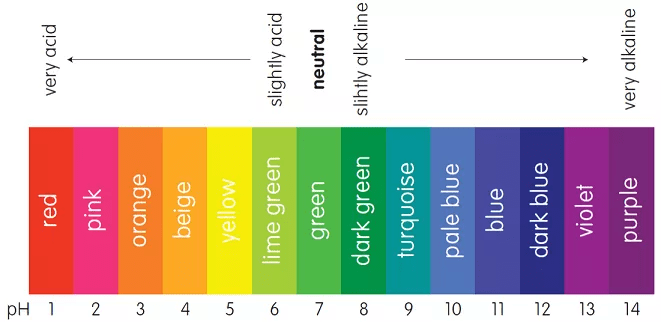pH scale used as indicatorThere are the substances, the by a sharp colour change, indicate the completion of a chemical reaction. Indicators for titration of acids and bases are usually weak organic acids or bases, yielding ions of a different colour from the unionized molecule, e.g., litmus is red with acids and blue with alkalies, a
change in colour indicates that neutralizations is complete.
On the basis of different titrations, indicators are classified into several types, viz.,

(a) neutralisation indicators (acid-base or pH indicators,

(b) oxidation-reduction indicators,

(d) mixed indicators,

(e) screened indicators,

(f) metal indicators and

(g) universal indicators

Double Indicators Titrations: For the titration of alkali mixtures (e.g., NaOH + Na2CO3) or (Na2CO3 + NaHCO3), two indicators phenolphthalein and methyl orange are used. Phenolphthalein is a weak organic acid and gives end point between pH 8 to 10, while methyl orange, a weak base itself indicatesend point sharply between pH 3.1 to 4.4. Following points must therefore, be remembered.

•  Phenolphthalein is not a good indicator for a weak alkali titrations.
•  Methyl orange is not a good indicator for weak acid titrations.
• In case of strong acid vs. Strong base titrations using phenolphthalein or methyl orange as indicator, the equivalent point suggests that meq. Of acid = meq. Of alkali.

In case of strong acid vs. Strong base such as Na2CO3 (which acts as strong base in I step of dissociation and weak base in II step dissociation), using phenolphthalein as indicator, the meq. Of acid are titrated only upto NaHCO3 state.
Na2CO3 +  H+ → NaHCO3 + Na+
weak
i.e., meq. Of acid = 1/2 meq. Of Na2CO3
(if Eq. Mass of Na2CO3 = M/2)

Or meq. Of acid = meq. Of Na2CO3
(if Eq. Mass of Na2CO= M/1)
However, titration with methyl orange as indicator, the meq. Of acid corresponds of total meq. Of alkali present at that time in mixture.

• One should be careful in calculating meq. Of alkalies, if methyl orange is used as indicator in titration, i.e., for fresh mixture or in continuation of phenolphthalein, e.g.,

Na2CO3 + NaOH mixture v.s HCl

Case I: I end point determined using phenolphthalein as indicator and then methyl orange is used to get II end point in continuation.
Case II: End point is determined using phenolphthalein as indicator. Next time end point is determined by taking another (fresh) same volume of mixture using methyl orange as indicator.

For Phenolphthalein:
Meq. Of Acid = meq. Of NaOH = 1/2 meq. Of Na2CO3

For Methyl Orange: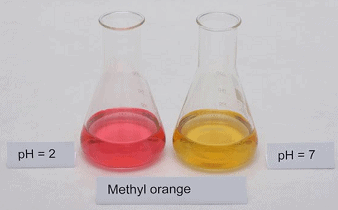Different colors of methyl orange at different pHMeq. Of acid = meq. Of NaOH = meq. Of Na2CO3

Example: 200 mL of a solution of mixture NaOH and Na2CO3 was first titrated with phenolphthalein and N/10 HCl. 17.5 mL of HCl was required for the end point. After this methyl orange was added and 2.5 mL of same HCl was again required for next end point. Find out the mass of NaOH and Na2CO3 in mixture:

Solution: Phenolphthalein as indicator:
Meq. Of HCl used for 200 mL solution = 17.5 x (1/10) = 1.75
∴ meq. Of NaOH + 1/2 meq. Of Na2CO3 = 1.75
Methyl Orange as indicator: After I end point, methyl orange is added
1/2 meq. Of Na2CO= meq. Of HCl used

= 2.5 x (1/10) = 0.25

By Eqs.
Meq. Of NaOH = 1.75 – 0.25 = 1.50
∴ (w/40) x 1000 = 1.50
Or WNaOH = 0.06 g per 200 mL
Also, meq. Of Na2CO3 = 0.25 x 2 = 0.50
∴ (w/53) x 1000 = 0.50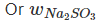= 0.0265 g per 200mL

Eudiometry

The technique involving volume measurement during the reaction of gases is called eudiometry. Since volume of gas, V ∝ number of mole at constant P, T and thus, volume ratio of gases can be directly used in place of mole ratio to analyse the given data.

Example: A mixture of ethane (C2H6) and ethene (C2H4) occupies 40 litre at 1.00 atm and at 400 K. The mixture reacts completely with 130 g of Oto produce CO2 and H2O. Assuming ideal gas behaviour, calculate the mole fractions of C2H4and C2H6 in the mixture.

Solution: For a gaseous mixture of C2H6 and C2H4
PV = nRT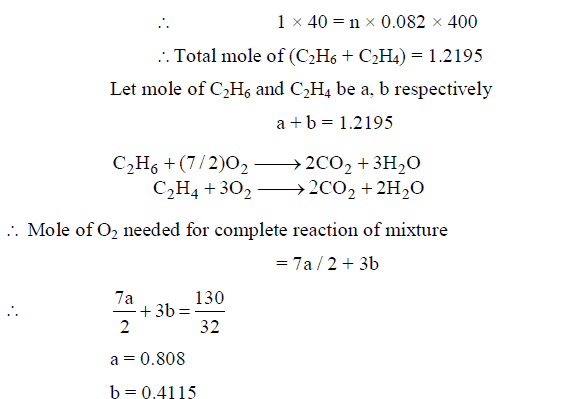∴ Mole fraction of C2H6 = 0.808 / 1.2195 = 0.66

And Mole fraction of C2H= 0.34

To represent concentration of H2Osolution:

In percentage: The mass of H2O2 present in 100 mL H2Osolution is H2O2 concentration in percentage of H2O2 solution.

In volume: The volume of Oat STP given by 1 mL H2Osolution on decomposition is H2Oconcentration of H2O in volume.
Direct conversions can be made by using following relations

• % strength 17/56 x  volume strength
• Volume strength = 5.6 x Normality
• Volume strength = 11.2 x Molarity

To represent the concentration of Oleum:
(100 + X%) of oleum means ‘X’ g H2O reacts with equivalent amount of free SO3 to give H2SO4.

Example: Calculate the % of free SO3 in oleum (a solution of SO3 in H2SO4) that is labelled 109% H2SO4)

Solution: percentage above 100 represents the mass of H2O that reacts with dissolved SO3 in oleum to give H2SO4, i.e., 9 g H2O reacts with free SOto produce H2SO4

18 g H2O reacts with 80 g SO3

∴9 g H2O will react with 40 g SO3
Or % of free SO3 = 40

To determine Hardness of water:

• Water, which gives foams easily with soap is called soft water and if not then hard water.
• The hardness of water is due to the presence of bicarbonates, chlorides and sulphates of Ca and Mg.
• The hardness is temporary due to bicarbonates and permanent due to chlorides and sulphates of Ca and Mg
• The extent of hardness is known as degree of hardness defined usually as the number of parts bymass of CaCO3  present per million parts by mass of water:
• Hardness is expressed in ppm i.e., 1 ppm = 1 part of CaCOin 106 part of hard water.
• The reason for choosing CaCO3 as the standard to express hardness, inspite of the fact that CaCO3 is not soluble in water but its molar mass is 100 which makes calculation easy.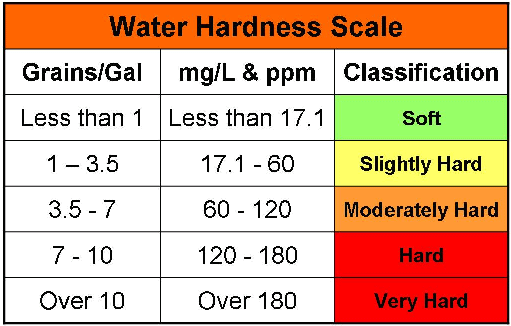Water hardness scale

Water softners: The hardness may be removed by either of the reactants on treating with water.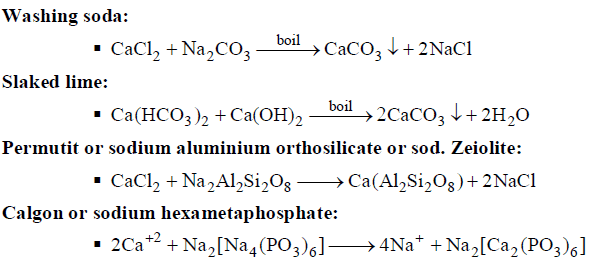Offer running on EduRev: Apply code STAYHOME200 to get INR 200 off on our premium plan EduRev Infinity!

## Physical Chemistry

77 videos|83 docs|32 tests

,

,

,

,

,

,

,

,

,

,

,

,

,

,

,

,

,

,

,

,

,

;